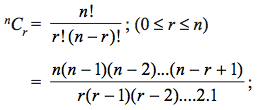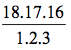In this section, the following topics are discussed with examples

• Counting formula for Combinations
• Important Rules
• Examples

### 1. Selection of Objects Without Repetition

The number of combinations or selections of different things taken at a time is denoted by nCr or (nr) where ,where is a natural number and is a whole number.

### SOME IMPORTANT RULES:## It is the combination of reasonable talent and the ability to keep going in the face of defeat that leads to success.

Sherry Bebitch Jeffe

#### Example 1:

If 20Cr 20Cr – 10, then find the value of 18Cr  .

Solution:

20Cr 20C– 10 ⇒ + (– 10) = 20 ⇒ = 1

∴ 18Cr 18C15 = 18C3= 816.

#### Example 2

How many different 4-letter words can be formed with the letters of the word ‘JAIPUR’ when and are always to be included ?
Solution:

Since and are always to be included, so first we select 2 letters from the remaining 4, which can be done in 4C2 = 6 ways. Now these 4 letters can be arranged in 4! = 24 ways, so the required number = 6 × 24 = 144.

### 2. Selection of Objects With Repetition

The total number of selections of r things from n different things when each thing may be repeated any number of times is n + r – 1Cr .

### 3. Restricted Selection

(i) Number of combinations of different things taken at a time when particular things always occur is – kC– k .

(ii) Number of combinations of different things taken at a time when particular things never occur i  – kCr.

### 4. Selection From Distinct Objects

Number of ways of selecting at least one thing from different things is
nC1 + nC2 + nC3 + …..+ nCn = 2n – 1.
This can also be stated as the total number of combination of different things is 2n – 1.